## General Question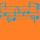# Do you think the transitive property of equality is superfluous when we have the substitution property?

Asked by dxs (15091) December 1st, 2014

There exists both a transitive property and a substitution property of equality. I think that having both of them is redundant, and I favor the substitution property over the transitive property.
The transitive property of equality states that If a=b & b=c, then a=c.
The substitution property of equality states that if a=b, then a & b can be substituted for each other in any expression.
Source

It seems as though whenever the transitive property is applied, it is implicitly the substitution property. So, why go out of the way to label it as its own property?

Observing members: 0Composing members: 0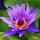Items involved in Transitive are THREE only TWO in substitution.

Tropical_Willie (26809)“Great Answer” (2) Flag as…@Tropical_Willie Not necessarily. For instance, let a=2 and a+b=5 We could write this as “If 2=a, and a=5-b, then 2=5-b. So the “c” part is 5-b. This can extend to however many equalities you want to make. (a1=a2, a2=a3, a3=a4, ... , av(n-1)=an ⇒ a1=an)

dxs (15091)“Great Answer” (0) Flag as…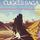Given: [(a = b) & (b = c)]—> (a = c)

Prove: (a = b)—> [ F(ab) <=> F(ac)] , where F denotes any predicate/relation/function (pretend it’s the Greek letter phi).

Or vice versa.

CugelTheClueless (1534)“Great Answer” (1) Flag as…Oops, that last bit should be . . . [F(ac) <=> F(bc)]

CugelTheClueless (1534)“Great Answer” (1) Flag as…The way I understand it, although the substitution property seems intuitively obvious, there is no such property at the fundamental levels of mathematics. What you have are a collection of properties like a=b => a+c=b+c and a=b => a*c=b*c. Suppose you have a=b and c=d. How do you show that a+c=b+d? We have a+c=b+c and also c+b=d+b. Using transitivity multiple times and the commuative law, we get a+c = b+c = c+b = d+b = b+d and therefore a+c = b+d. I suppose that, with a bit of effort, you could use the fundamental laws to derive a general substitution property.

LostInParadise (27920)“Great Answer” (1) Flag as…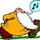@CugelTheClueless Here is a cut-and-paste Greek letter phi

ϕ

So that last equation should be [ϕ(ac) <=> ϕ(bc)]

Strauss (21179)“Great Answer” (1) Flag as…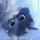Well, I’m only a high school student, currently learning Geometry…so I’m not sure my opinion is going to amount to much. But, I think its useful for high school students when they are starting to use these properties more, for example in proofs for Geometry. Its just more specific. And, for myself and other like me, when you’re not the greatest math whiz its helpful.

But, I understand why its kinda redundant. Its similar to the substitution property and the symmetric property to me.

LeilaniLane (181)“Great Answer” (1) Flag as…Sorry I haven’t responded. Finals are around the corner so I’ve been busy with school.
@LeilaniLane I loved Geometry! Proofs were my favorite. I remember always being stuck on the difference between substitution and transitive. This is the case I’m talking about where it seems like they’re interchangeable.
Look at this geometry worksheet I found online. Look at problem 3 vs. problem 7. Could one use say that in #3 they executed the last step using transitivity and in #7 using substitution? This kind of grey area is what I was targeting when I originally posted this question.
@LostInParadise That’s it! So really, substitution is more complex than the reflexive, symmetric, and transitive properties. Substitution seems to define equality.

dxs (15091)“Great Answer” (0) Flag as…@dxs , This Wikipedia article may be a little advanced, but try looking at the Formulation section. It shows how natural numbers can be defined by a few axioms. Basically what it says is that the defining property of natural numbers is that they can be counted. That is the idea of the successor function. What is extraordinary (I majored in math, so I may be a tad biased) is that it is possible from this small set of axioms to define all the arithmetic operations and do the proofs for them. It is then possible to define integers (positive and negative) as an extension of natural numbers and successively create extensions for rationals, reals and complex numbers and prove the basic properties. This was a major accomplishment of 19th century mathematics.

LostInParadise (27920)“Great Answer” (1) Flag as…@dxs Haha. Well at least one of us does… or did. I really dislike any form of math. Currently, I’m staring at my Geometry assignment thinking, “why?” Its my last course before I’m done with this segment. Yeah, I was thinking that was what you meant.

Strangely that looks similar to what they ask me to do on my quizzes. I guess I understand what you mean. I think that’s addressed here actually: http://mathforum.org/library/drmath/view/64446.html

LeilaniLane (181)“Great Answer” (2) Flag as…I’m pretty sure that’s what LostInParadise was saying… its just easier for me to understand since it has more written explanation then… math equations.

LeilaniLane (181)“Great Answer” (0) Flag as…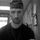Yes, I agree with your remarks in the question.

Sinqer (520)“Great Answer” (1) Flag as…or